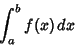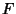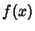## Definite Integral

An Integralwith upper and lower limits. The first Fundamental Theorem of Calculus allows definite integrals to be computed in terms of Indefinite Integrals, since ifis the Indefinite Integral for, then